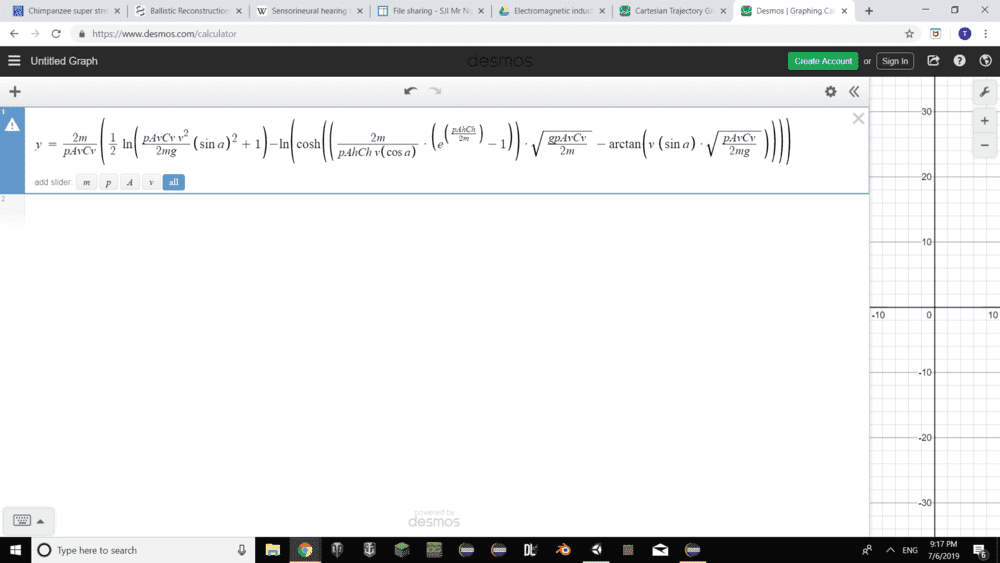# Problem with an Equation -- Solving for "a" in this large equation

Ok, I know this may sound silly and I apologize for bringing in what should be a relatively easy equation. I'm trying to make the small a in the below equation the subject of the formula (That is, to push everything in the equation except a to one side of the equation and leave all the small letter a on the other side), but I'm getting stuck trying to do so halfway:

(Note: I'm trying to make the small letter a the subject of the formula, NOT the capital letter A's!)PS: The small dots you see in the equation are multiplication operators. They just mean this multiplied by this

Would appreciate any help for this! :)

Last edited by a moderator:
•Buzz Bloom

## Answers and Replies

jedishrfu
Mentor
You could replace the many factors with a composite factor to reduce the equation to something a little more manageable:

##y = A ( (1/2)* ln ( B * sin^2(a) + 1) - ln ( cosh ( C / cos(a) ) * D - arctan ( v * sin(a) * E ) ) ) )##

where:
Code:
A = ...
B = ...
C = ...
D =  ( e^(...) - 1 )
E = ...

•TheShermanTanker
Buzz Bloom
Gold Member
Hi TheShemanTanker:

I think that Jedishrfu's suggestion is a good first step, although I would also eliminate v, including it in E. I suggest the following as a second step.

Take the exponential of both sides.

ey = . . .

Hope this helps.

Regards,
Buzz

•TheShermanTanker